Types of Functions in Precalculus Chapter Exam

Exam Instructions:

Choose your answers to the questions and click 'Next' to see the next set of questions. You can skip questions if you would like and come back to them later with the yellow "Go To First Skipped Question" button. When you have completed the practice exam, a green submit button will appear. Click it to see your results. Good luck!

Page 1

Question 5 5. Choose the answer that best describes the function of this graph.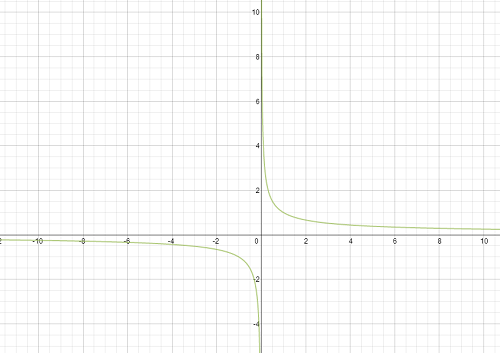Page 2

Question 8 8. Using the function below, evaluate g(7).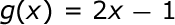Question 9 9. Choose the answer that best describes the function of this graph. Select the best answer choice that describes the function of this graph.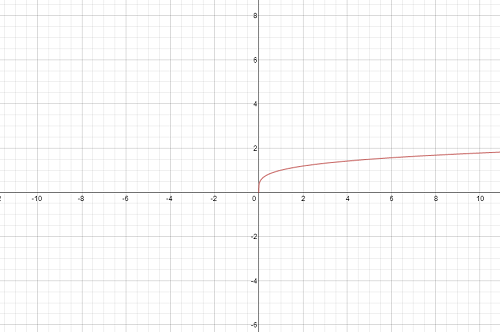Page 3

Question 12 12. Choose the answer that best describes the function of this graph.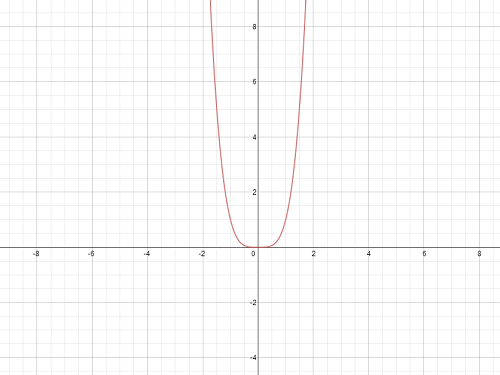Question 14 14. Which equation matches the following graph?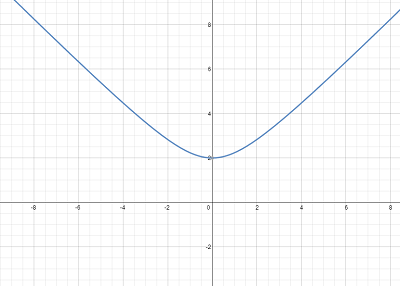Question 15 15. Which equation matches the graph?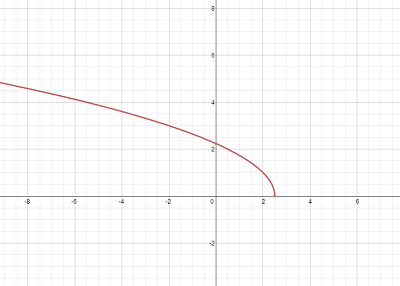Page 4

Question 17 17. What is the range of the function represented by the graph below?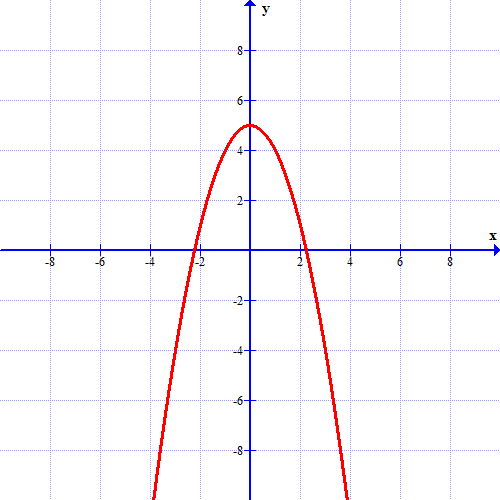Question 19 19. What is the domain of the function below?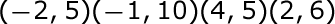Question 20 20. Choose the answer that best describes the function of this graph. Look at the graph that is shown below. Which answer choice best describes the function of this graph?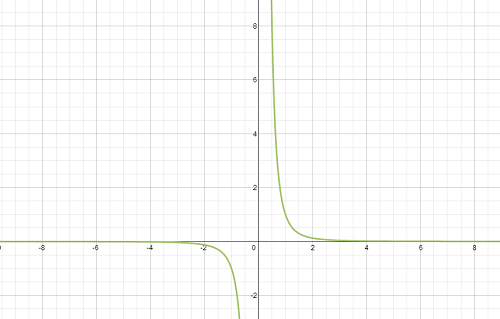Page 5

Question 23 23. Choose the answer that best describes the function of this graph.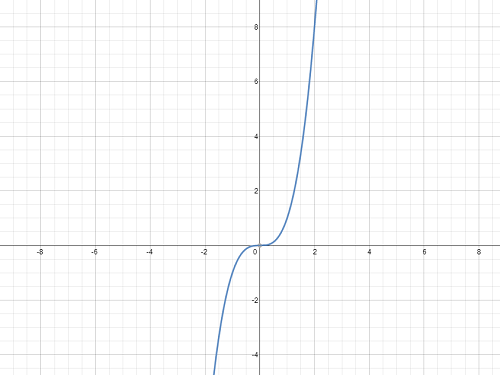Question 25 25. Which equation matches the following graph?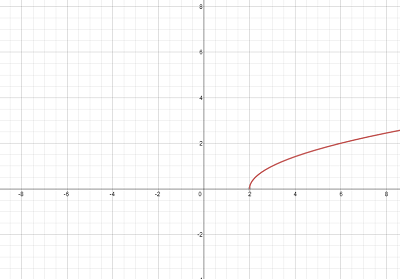Types of Functions in Precalculus Chapter Exam Instructions

Choose your answers to the questions and click 'Next' to see the next set of questions. You can skip questions if you would like and come back to them later with the yellow "Go To First Skipped Question" button. When you have completed the practice exam, a green submit button will appear. Click it to see your results. Good luck!

Support